Question

# 8. The cantilever beam in Figure Q8 subjects to concentrated loading. The cross section geometry gives...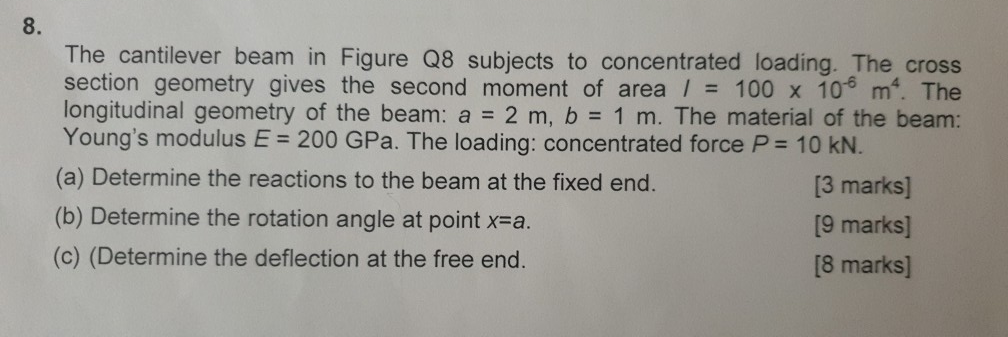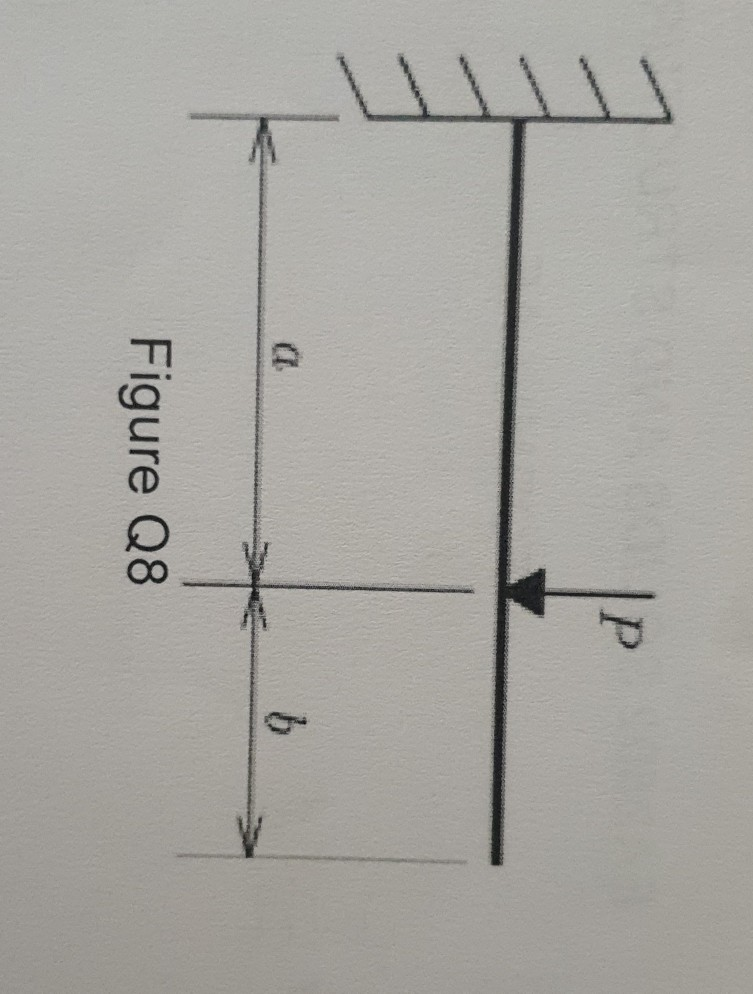8. The cantilever beam in Figure Q8 subjects to concentrated loading. The cross section geometry gives the second moment of area / 100 x 10 m. The longitudinal geometry of the beam: a 2 m, b 1 m. The material of the beam: Young's modulus E 200 GPa. The loading: concentrated force P 10 KN. (a) Determine the reactions to the beam at the fixed end. (b) Determine the rotation angle at point x-a (c) (Determine the deflection at the free end. 6 4 [3 marks] [9 marks] [8 marks]
Figure Q8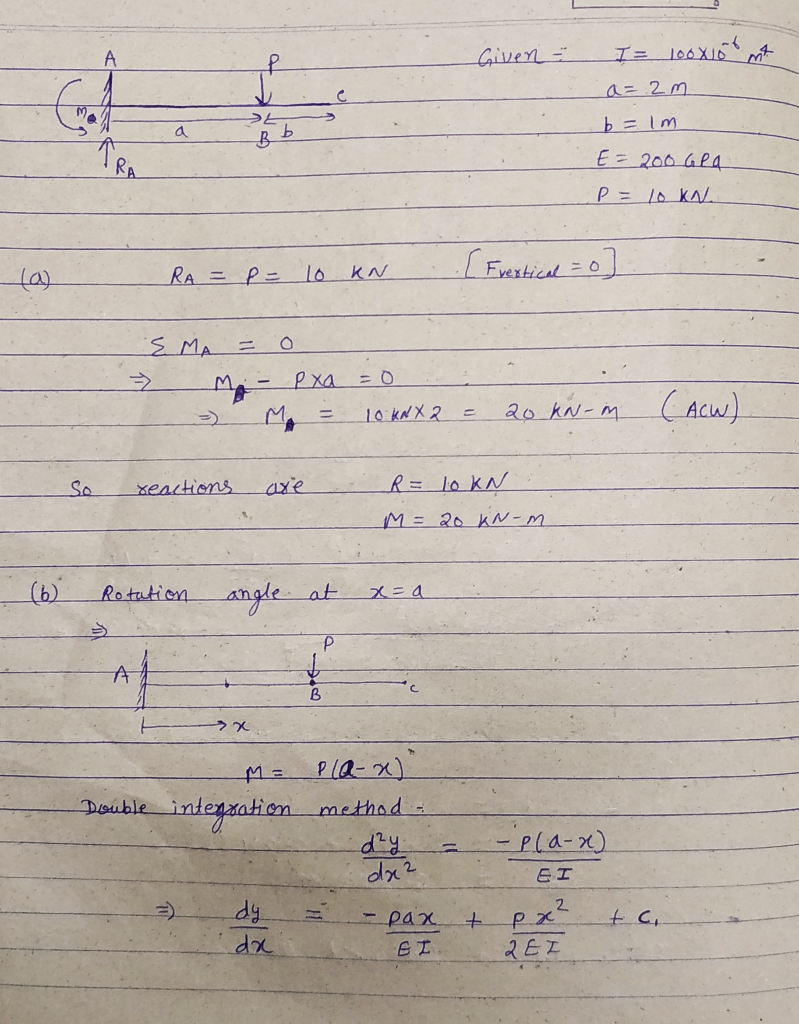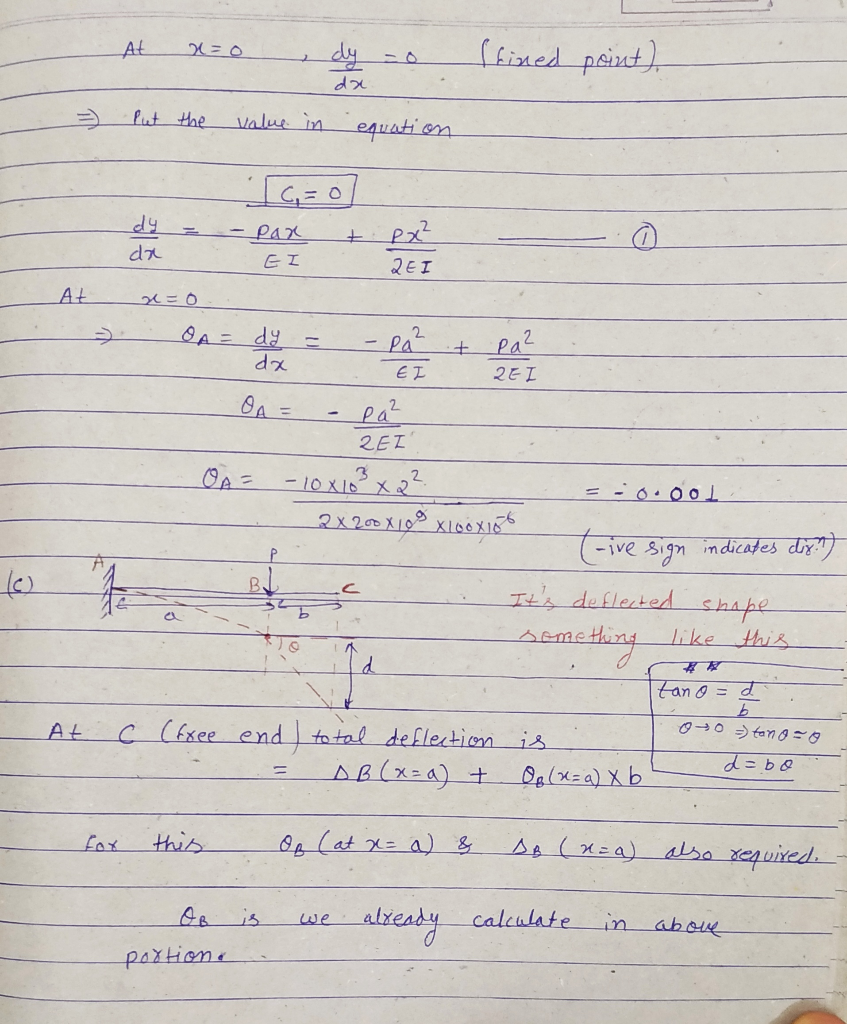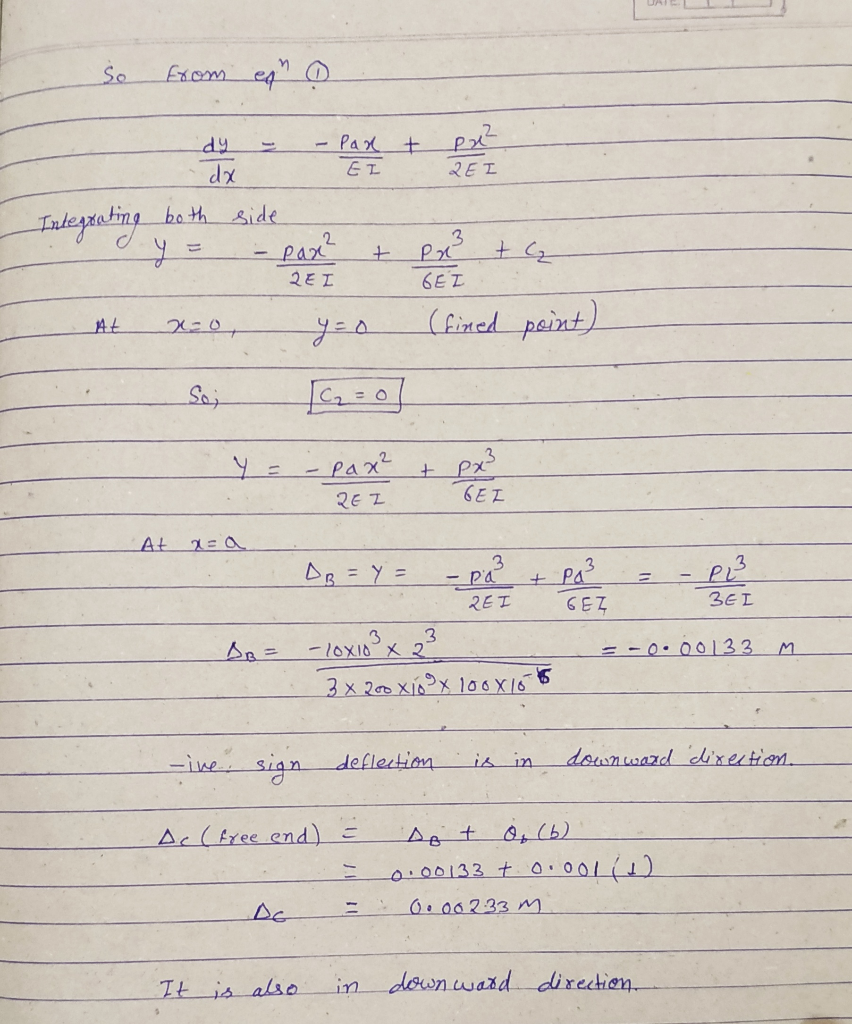#### Earn Coins

Coins can be redeemed for fabulous gifts.

Similar Homework Help Questions
• ### Q5. The cantilever beam, AC, is subjected to the load case shown in Figure 5. For the loading shown, do the following: [10 Marks] a) Calculate the magnitude and direction of the reactions at A b) Usi...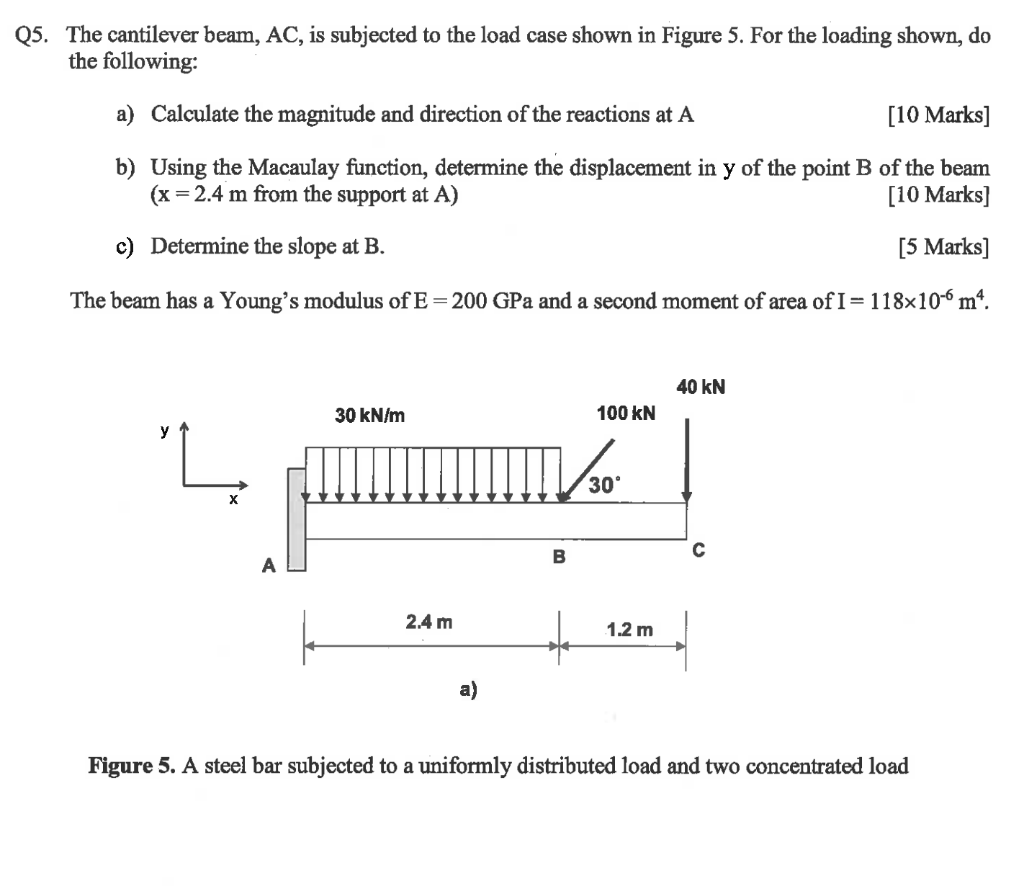Q5. The cantilever beam, AC, is subjected to the load case shown in Figure 5. For the loading shown, do the following: [10 Marks] a) Calculate the magnitude and direction of the reactions at A b) Using the Macaulay function, determine the displacement in y of the point B of the beam (x 2.4 m from the support at A) [10 Marks] c) Determine the slope at B. [5 Marks] The beam has a Young's modulus of E-200 GPa and...

• ### A cantilever beam AB is subjected to a triangle loading with concentrated moment (see figure). The...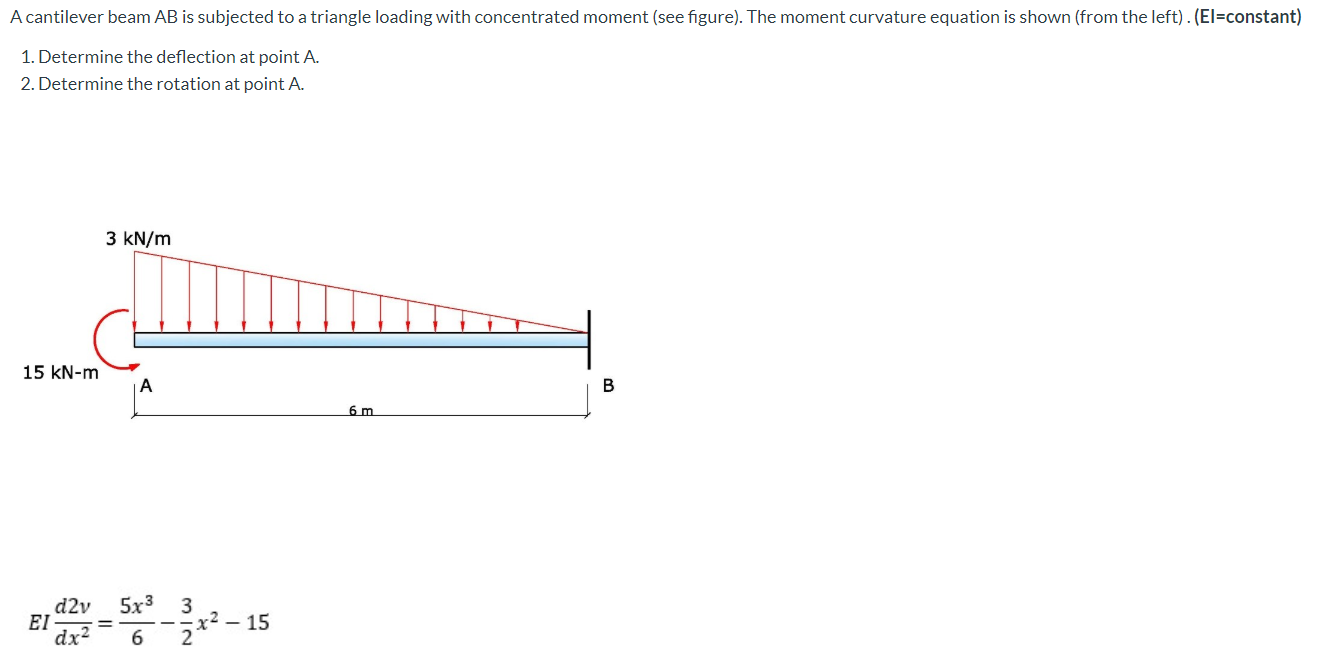A cantilever beam AB is subjected to a triangle loading with concentrated moment (see figure). The moment curvature equation is shown (from the left). (El=constant) 1. Determine the deflection at point A. 2. Determine the rotation at point A. 3 kN/m 15 kN-m A B 6 m d2v ΕΙ 5x3 3 x2 – 15 2 dx2 6

• ### A cantilever beam of a channel section is loaded at its half-length, as shown in Figure Q2. The Young's modulus of the material is 200 GPa. Determine the deflection at the free end. [12.5 marks]...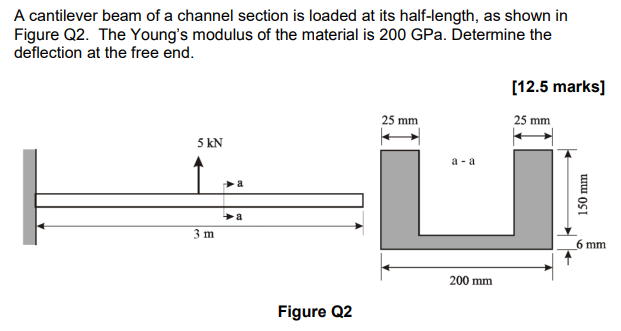A cantilever beam of a channel section is loaded at its half-length, as shown in Figure Q2. The Young's modulus of the material is 200 GPa. Determine the deflection at the free end. [12.5 marks] 25 mm 25 mm 5 kN a -a 少a 6 mm 200 mm Figure Q2 A cantilever beam of a channel section is loaded at its half-length, as shown in Figure Q2. The Young's modulus of the material is 200 GPa. Determine the deflection at...

• ### Q1 An elastic cantilever beam of varying cross section, as shown in Figure Q1(a), is subjected...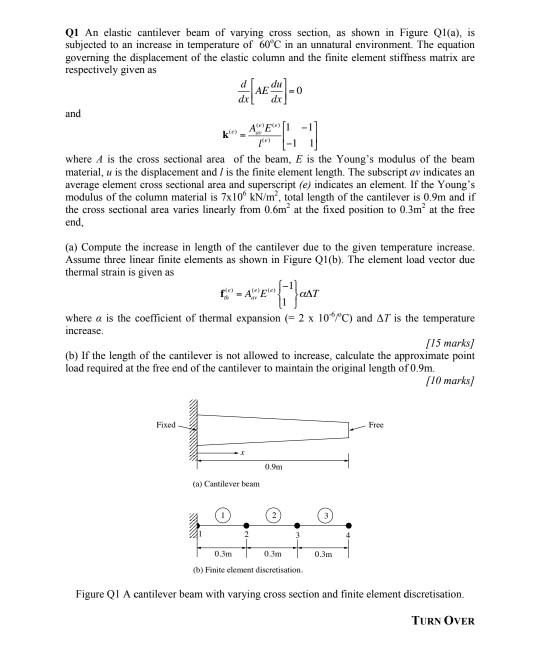Q1 An elastic cantilever beam of varying cross section, as shown in Figure Q1(a), is subjected to an increase in temperature of 60°C in an unnatural environment. The equation governing the displacement of the elastic column and the finite element stiffness matrix are respectively given as -O and ΑΕ) - where A is the cross sectional area of the beam, E is the Young's modulus of the beam material, u is the displacement and / is the finite element length....

• ### Acantilever beam AB is subjected to a triangle loading with concentrated moment (see figure). The moment...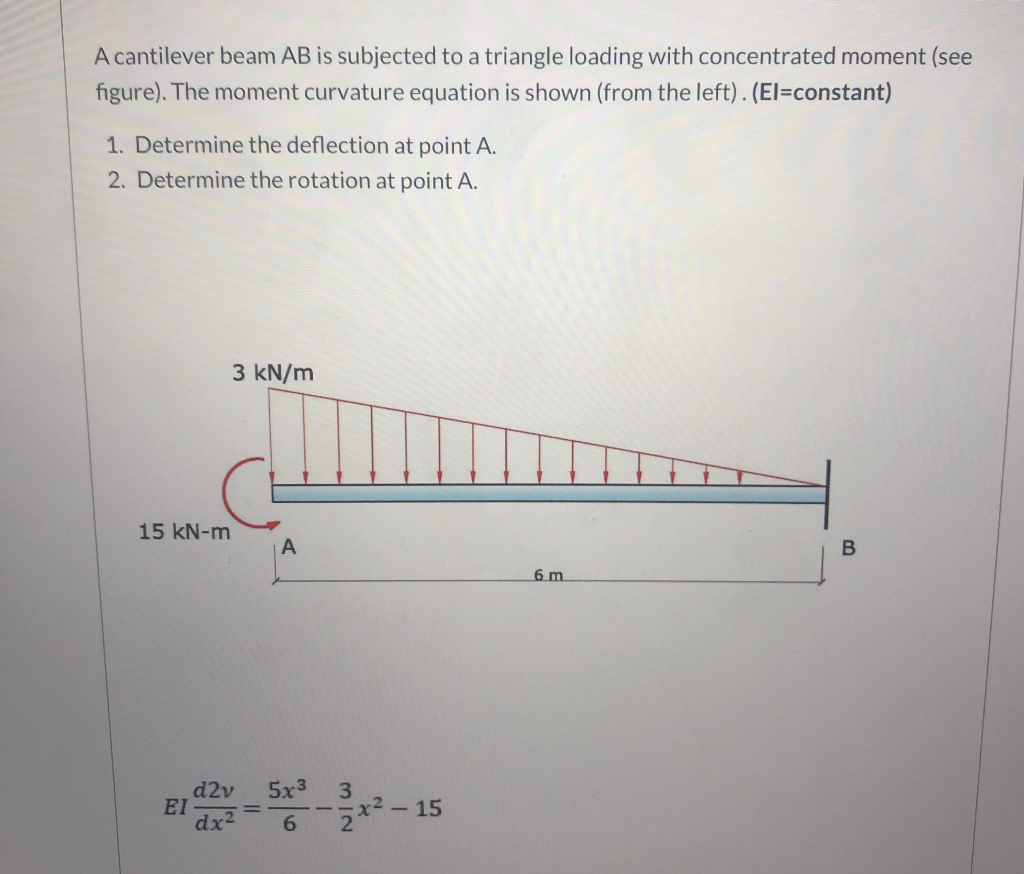Acantilever beam AB is subjected to a triangle loading with concentrated moment (see figure). The moment curvature equation is shown (from the left). (El=constant) 1. Determine the deflection at point A. 2. Determine the rotation at point A. 3 kN/m 15 kN-m B 6 m d2v EI dx- 5x3 3 --x2 - 15 6 2

• ### Q1. For the cantilever beam and loading shown with circular section of 60 mm diameter and...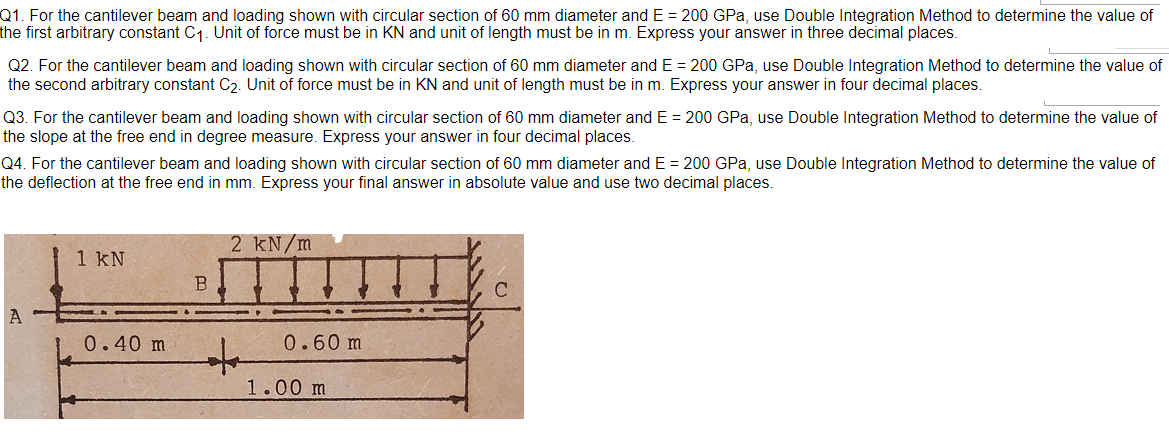Q1. For the cantilever beam and loading shown with circular section of 60 mm diameter and E = 200 GPa, use Double Integration Method to determine the value of the first arbitrary constant C1. Unit of force must be in KN and unit of length must be in m. Express your answer in three decimal places. Q2. For the cantilever beam and loading shown with circular section of 60 mm diameter and E = 200 GPa, use Double Integration Method...

• ### The cantilever beam AC with length L=5 m has elasticity modulus E= 172 GPa, cross-section with...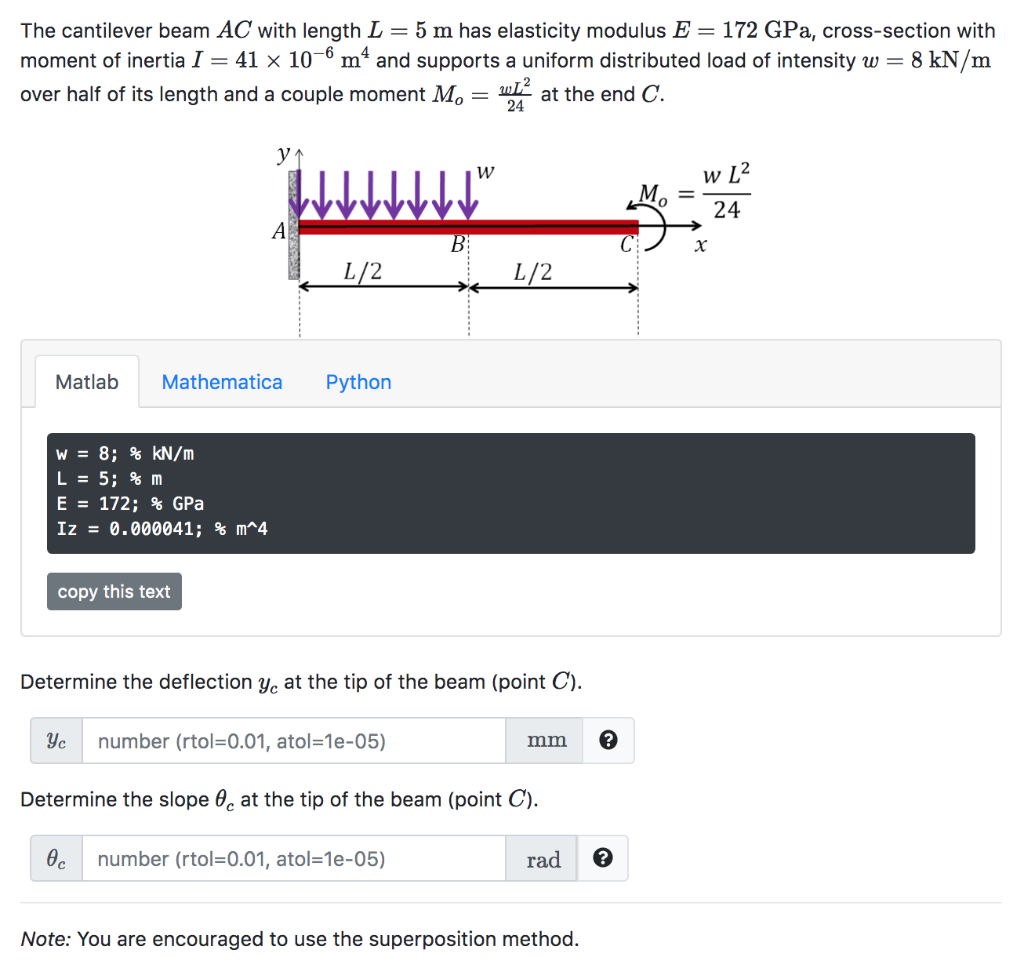The cantilever beam AC with length L=5 m has elasticity modulus E= 172 GPa, cross-section with moment of inertia I = 41 x 10-6 mº and supports a uniform distributed load of intensity w = 8 kN/m over half of its length and a couple moment Mo = win at the end C. W w L2 VV WWWV В x 1 1/2 1/2 C) . Matlab Mathematica Python W = 8; % kN/m L = 5;%m E = 172; %...

• ### Question 3 For the simply supported steel beam with cross section and loading shown (see Figure...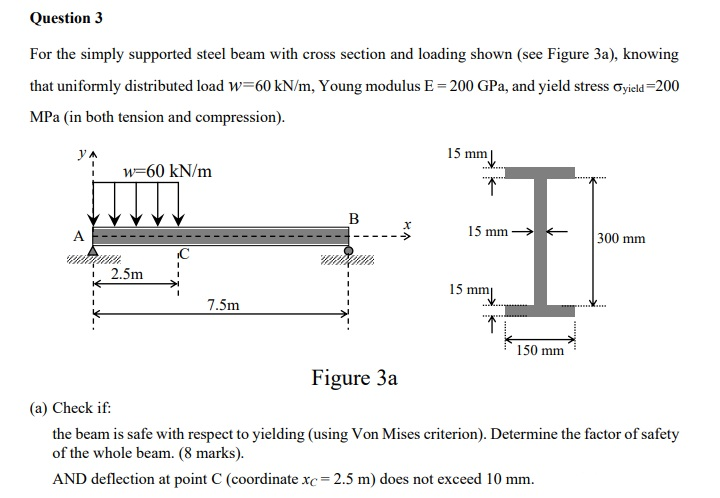Question 3 For the simply supported steel beam with cross section and loading shown (see Figure 3a), knowing that uniformly distributed load w=60 kN/m, Young modulus E = 200 GPa, and yield stress Cyield=200 MPa (in both tension and compression). ул 15 mm w=60 kN/m ... 1 B A 15 mm + 300 mm IC - i 2.5m 1 1 15 mm 7.5m 1 150 mm Figure 3a (a) Check if: the beam is safe with respect to yielding (using...

• ### The cross section of the cantilever beam loaded as shown in Fig. 8-20 is rectangular, 50 × 75 mm. The bar, 1 m long, i...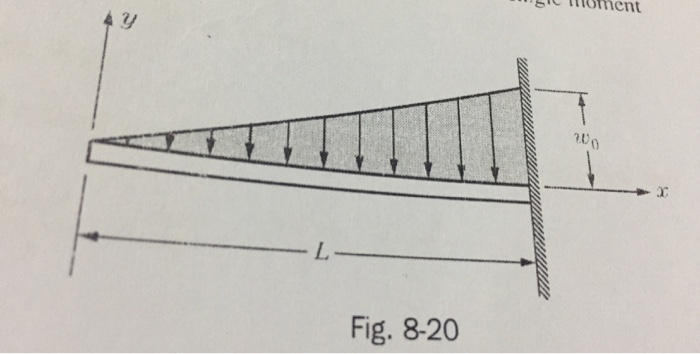The cross section of the cantilever beam loaded as shown in Fig. 8-20 is rectangular, 50 × 75 mm. The bar, 1 m long, is aluminum for which E = 65 GPa. Determine the permissible maximum intensity of loading if the maximum deflection is not to exceed 5 mm and the maximum stress is not to exceed 50 MPa. Ans. w0 = 14.1 kN/m and 17.1 kN/m. Select 14.1 kN/m. oment 3 Fig. 8-20 oment 3 Fig. 8-20

• ### Check my work For the cantilever beam and loading shown, determine the slope and deflection at...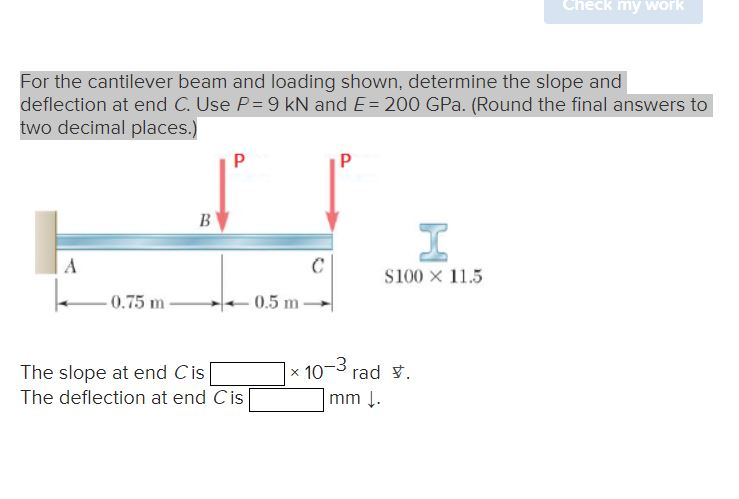Check my work For the cantilever beam and loading shown, determine the slope and deflection at end C. Use P = 9 kN and E= 200 GPa. (Round the final answers to two decimal places.) P Р B I A \$100 X 11.5 -0.75 m 0.5 m The slope at end Cis The deflection at end Cis x 10m rad . -3 mm.